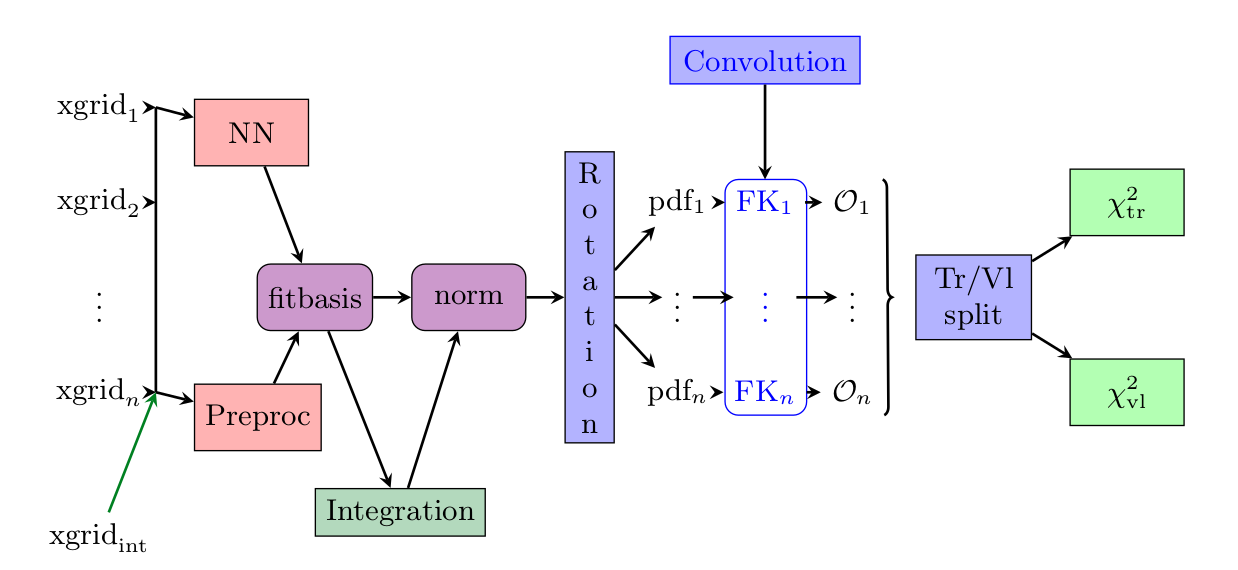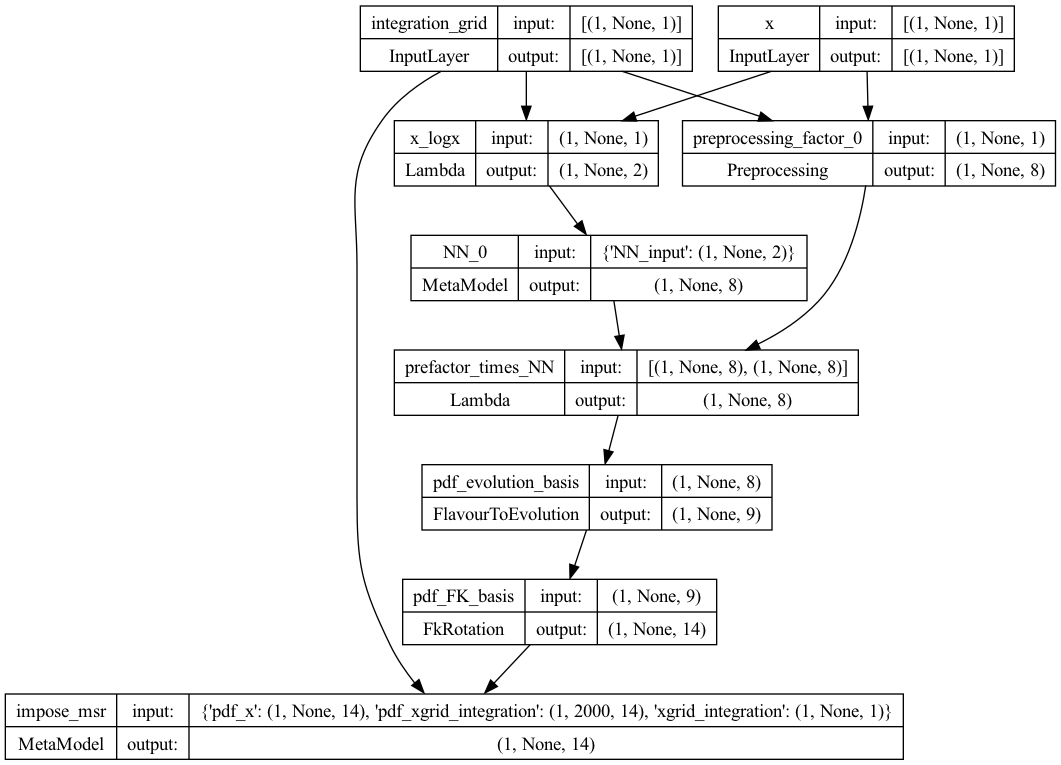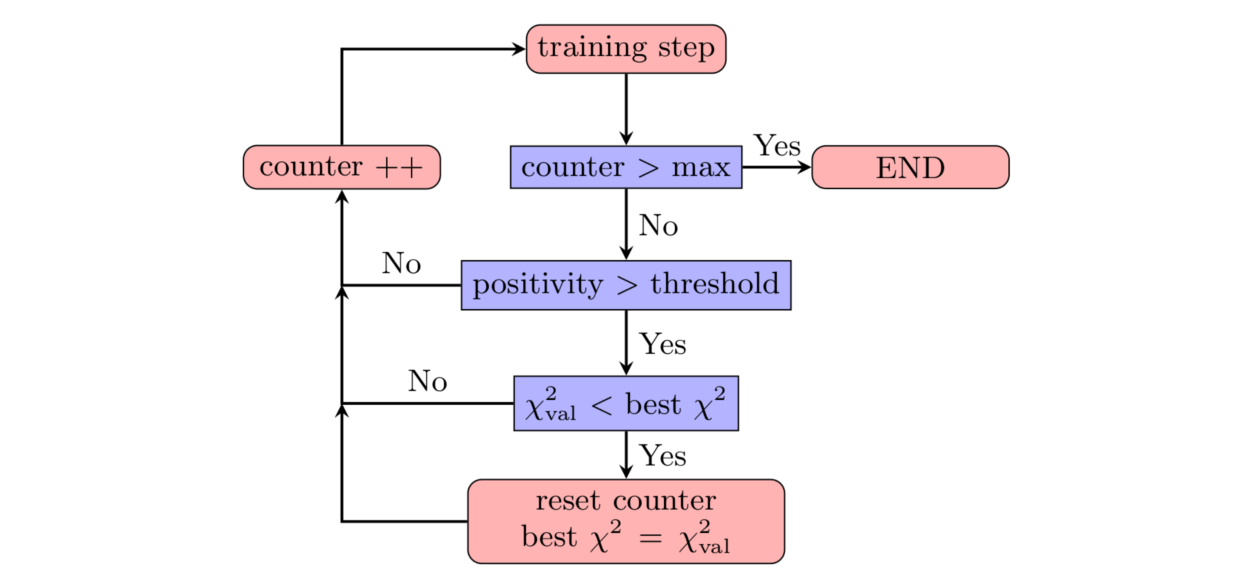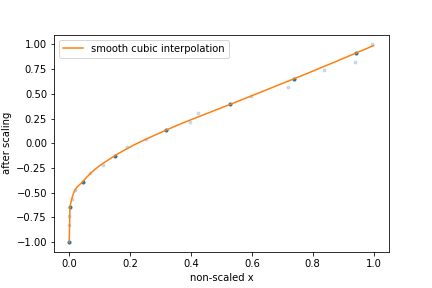# Methodology overview

The goal of this document is to summarise from a conceptual point of view the main points which are different in comparison to the latest NNPDF (i.e. NNPDF3.1) methodology.

Warning

The default implementation of the concepts presented here are implemented with Keras and Tensorflow. The n3fit code inherits its features, so in this document we avoid the discussion of specific details which can be found in the Keras documentation.

Note

The final setup used in n3fit fits can be extracted from the runcards stored in nnpdf/n3fit/runcards.

This document contains a more specific discussion about the choices currently implemented in the n3fit package and discussed for the first time in hep-ph/1907.05075.

## Introduction

The approach presented here inherits the technology developed by the NNPDF collaboration in terms of fit pipeline but extends the possibility to test and improve fitting performance with modern techniques inspired by the deep learning community.

The n3fit code is designed in python and replaces the nnfit program used in the NNPDF3.X family of fits. It provides a simple abstraction layer which simplifies the life of developers when considering the possibility of adding new fitting algorithms.

In the following table we list some of the differences between both codes:

Component

nnfit

n3fit

Random numbers

main seed, closure filter seed

multi seed

Data management

libnnpdf

same as nnfit

Neural net

fixed architecture, per flavour

single net, flexible architecture

Preprocessing

random fixed

fitted in range

Integration

a posteriori per iteration

built into in the model

Optimizer

genetic optimizer

Stopping

lookback

patience

Positivity

penalty and threshold

dynamic penalty, PDF must fulfill positivity

Postfit

4-sigma chi2 and arclength

same as nnfit

Fine tuning

manual

semi-automatic

Model selection

closure test

closure test, hyper optimization

Input scaling

(x,log(x))

feature scaling

In nnfit there is a single seed variable stored in the fit runcard which is used to initialize an instance of the RandomGenerator class which provides random numbers sequentially. The nnfit user has no independent control over the random number sequences used for the neural network initialization, the training-validation split and the MC replica generation. On the other hand, in n3fit we introduce three new seed variables in the fit runcard: trvlseed for the random numbers used in training-validation, nnseed for the neural network initialization and mcseed which controls the MC replica generation.

Note

In the next sections we focus on the n3fit specifics marked in bold.

## Neural network architecture

The main advantage of using a modern deep learning backend such as Keras/Tensorflow consists in the possibility to change the neural network architecture quickly as the developer is not forced to fine tune the code in order to achieve efficient memory management and PDF convolution performance.

The current n3fit code supports feed-forward multilayer perceptron neural networks (also known as sequential dense networks in ML code frameworks) with custom number of layers, nodes, activation functions and initializers from Keras.

A big difference in comparison to nnfit is the number of neural networks involved in the fit. Here we use a single neural network model which maps the input (x, log x) to 8 outputs, nominally they correspond exactly the 8 PDF flavours defined in NNPDF3.1. Note however that n3fit also allows for the use for one network per flavour by modifying the layer_type parameter.Preprocessing has been modified from fixed random range selection to fitted preprocessing in a bounded range by constraining the exponents to have the norm between a lower bound and an upper bound. The preprocessing ranges are the same used in NNPDF3.1 and thus based on the evolution basis with intrinsic charm.

The momentum sum rules are implemented as a neural network layer which computes the normalization coefficients for each flavour. This layer approximates the integral with a sum over a fixed grid of points in x. This approach guarantees that the model will always be normalized, even if the network parameters are changed, and therefore the gradient descent updates are performed correctly. The number and density of points in x is selected in such way that the final quality of the integrals are at least permille level in comparison to 1D integration algorithms.

The network initialization relies on modern deep learning techniques such as glorot uniform and normal (see Keras initializers), which have demonstrated to provide a faster convergence to the solution.

Important

Parameters like the number of layers, nodes, activation functions are hyper-parameters that require tuning.

To see the structure of the model, one can use Keras’s plot_model function as illustrated in the script below. See the Keras documentation for more details.

from tensorflow.keras.utils import plot_model
from n3fit.model_gen import pdfNN_layer_generator
from validphys.api import API

fit_info = API.fit(fit="NNPDF40_nnlo_as_01180_1000").as_input()
basis_info = fit_info["fitting"]["basis"]

pdf_models = pdfNN_layer_generator(
nodes=[25, 20, 8],
activations=["tanh", "tanh", "linear"],
initializer_name="glorot_normal",
layer_type="dense",
flav_info=basis_info,
fitbasis="EVOL",
out=14,
seed=42,
dropout=0.0,
regularizer=None,
regularizer_args=None,
impose_sumrule="All",
scaler=None,
parallel_models=1,
)

pdf_model = pdf_models
nn_model = pdf_model.get_layer("NN_0")
msr_model = pdf_model.get_layer("impose_msr")
models_to_plot = {
'plot_pdf': pdf_model,
'plot_nn': nn_model,
'plot_msr': msr_model
}

for name, model in models_to_plot.items():
plot_model(model, to_file=f"./{name}.png", show_shapes=True)


This will produce for instance the plot of the PDF model below, and can also be used to plot the neural network model, and the momentum sum rule model.## Preprocessing

Preprocessing has been modified from fixed random range selection to fitted preprocessing in a bounded range. The preprocessing ranges are defined in the the same from NNPDF3.1 and are defined in the fitting:basis parameter in the nnpdf runcard.

The old behaviour, in which the preprocessing is fixed randomly at the beginning of the fit, can be recovered by setting the trainable flag to false. See the detailed runcard guide for more information on how to define the preprocessing.

## Optimizer

In n3fit the genetic algorithm optimizer is replaced by modern stochastic gradient descent algorithms such as RMS propagation, Adam, Adagrad, among others provided by Keras. The development approach adopted in n3fit includes the abstraction of the optimization algorithm thus the user has the possibility to extend it with new strategies. By default all algorithms provided by Keras are available, other algorithms can be used by implementing them in the appropiate backend.

Following the gradient descent approach the training is performed in iteration steps where:

• for each data point the neural network is evaluated (forward propagation)

• the accumulated errors of each parameter is computed using the backward propagation algorithm, where starting from the analytical gradient of the loss function as a function of the neural network parameters the errors for each parameter is estimated.

• each parameter is updated accordingly to its weight, the gradient direction and the gradient descent update scheme (which controls the convergence step size and speed).

The gradient descent schemes are usually controlled by the learning rate, and the total number of iterations. Examples of fits using the n3fit methodology are available here:

• DIS-only fit based on NNPDF3.1 NNLO setup: view

• Global fit based on NNPDF3.1 NNLO setup: view

Important

The gradient descent scheme (RMSprop, Adagrad, etc.), the learning rate, the number of iteractions are hyper-parameters that require tuning.

## Stopping algorithm

n3fit implements a patience algorithm which, together with the positivity constraints, define when a fit is allowed to stop:Following the diagram presented in the figure above, we then train the network until the validation stops improving. From that point onwards, and to avoid false positives, we enable a patience algorithm. This algorithm consists on waiting for a number of iterations before actually considering the fit finished. This strategy avoids long fits by terminating the fitting at early stages thanks to the patience tolerance.

If the patience is set to a ratio 1.0 (i.e., wait until all epochs are finished) this algorithm is equal to that used in nnfit.

The look-back approach implemented in nnfit is not required by n3fit due to its less stochastic/random path towards the solution.

Important

The patience and the lagrange multipliers are hyper-parameters of the fit which require specific fine tuning.

## Positivity

In NNPDF3.1 the positivity of a set of chosen DIS and fixed-target Drell-Yan processes was required: PDFs were allowed to be negative, as long as these physical cross sections resulted to be positive. Since $$\overline{MS}$$ PDFs have been proved to be positive it is now convenient to require positivity of the distributions $$q_k = \{u,\bar{u},d,\bar{d},s,\bar{s},g\}$$ themselves. In n3fit this is done on the top of the DIS and Drell-Yan processes already considered in nnfit.

The implementation of such positivity constraints is based on a penalty term controlled by a positivity multiplier: for each positivity observable $$\mathcal{O}_k$$ (which can now be either a PDF or a physical cross section) we add to the total $$\chi^2$$ a term of the kind

$\chi^2_{k,pos} = \Lambda_k \sum_i \Theta\left(-\mathcal{O}_k\left(x_i,Q^2\right)\right),$

where $$\Lambda_k$$ is the Lagrange multiplier associated with the positivity observable $$\mathcal{O}_k$$. The points $$x_i$$ are chosen in the whole $$x$$-region. More precisely, they consist of 10 points logarithmically spaced between $$5 \times 10^{-7}$$ and $$10^{-1}$$ and 10 points linearly spaced between 0.1 and 0.9. The scale at which positivity is imposed is taken to be $$Q^2 = 5\,GeV^2$$. During the minimization, fit solutions giving negative values of $$\mathcal{O}_k$$ will receive a positive contribution to the total $$\chi^2$$ and therefore will be penalized. A similar methodology was already used in nnfit, to impose positivity of DIS and Drell-Yan physical cross sections.

At the end of the fit, each n3fit replica is tagged with the flags POS_VETO or POS_PASS, according to whether or not each positivity penalty is greater than a given threshold, set equal to $$10^{-6}$$ (note that the value of this threshold was set differently in nnfit, where less stringent positivity requirements were implemented). The postfit selection only accepts replicas which pass all positivity constraints, i.e., only replicas tagged as POS_PASS are retained.

Note as well that the positivity penalty in n3fit grows dynamically with the fit to facilitate quick training at early stages of the fit.

## Integrability

In order to satisfy valence and Gottfried sum rules, the distributions $$q_k = V,V_3,V_8, T_3, T_8$$ have to be integrable at small-$$x$$. This implies that

$\lim_{x\rightarrow 0} x q_k\left(x,Q_0^2\right) = 0.$

Similarly to what is done for positivity, we can impose this behaviour by adding an additional term to the total $$\chi^2$$ which penalizes fit solutions where the integrable distributions do not decrease to zero at small-$$x$$. This term is

$\chi^2_{k,integ} = \Lambda_k \sum_i \left[x_i q_k\left(x_i,Q^2\right)\right]^2.$

The specific points $$x_i$$ used in this Lagrange multiplier term depend on the basis in which the fit is performed: when working in the evolution basis, integrability is already imposed through the choice of preprocessing exponents, and therefore a single small-$$x$$ point $$x=10^{-9}$$ is used; when working in the flavour basis, no small-$$x$$ preprocessing term is implemented, and therefore more stringent integrability conditions are used to enforce an integrable small-$$x$$ behaviour. In particular, the three small-$$x$$ points $$x_i = 10^{−5} , 10^{−4} , 10^{−3}$$ are used in the definition of the Lagrange multiplier term above. After the fit, the postfit script will retain just those replicas satisfying a given numerical definition of integrability, as documented in the postfit section.

It should be noted that the positivity and integrability multipliers are hyper-parameters of the fit which require specific fine tuning through hyper-optimization.

## Feature Scaling

Up to NNPDF4.0 the input to the neural network consisted of an input node (x), which in the first layer is transformed to (x,log(x)) before being connected to the trainable layers of the network. The choice for the (x,log(x)) split is motivated by the fact that the pdfs appear to scale linearly in the large-x region, which is roughly [1e-2,1], while the scaling is logarithmic in the small-x region below x=1e-2. However, gradient descent based optimizers are incapable of distinguishing features across many orders of magnitude of x, this choice of input scaling means that the algorithm is limited to learning features on a logarithmic and linear scale.

To solve this problem there is the possibility to apply a different feature scaling to the input by adding a interpolation_points: [number of points] flag to the n3fit runcard. By adding this flag the (x,log(x)) scaling is replaced by a scaling in such a way that all input x values are evenly distributed on the domain [-1,1], and the input node is no longer split in two.

Of course this scaling is discrete while the pdfs must be continuous. Therefore a monotonically increasing cubic spline is used to interpolate after the scaling has been applied. To this end the PchipInterpolator function from the scipy library is used. However, this way the neural network will be agnostic to the existence of this interpolation function meaning it can no longer learn the true underlying law. To fix this, the interpolation function has to be probed as well. This is done by only using [number of points] set by the interpolation_points flag to define the interpolation function after the scaling has been applied. Using this methodology the points used in the interpolation are again evenly distributed.The figure above provides a schematic representation of this feature scaling methodology:

1. The input x are mapped onto the [-1,1] domain such that they are evenly distributed.

2. [number of points] points are kept (dark blue), while other points are discarded (light blue).

3. A cubic spline function is used to do the interpolation between the points that have not been discarded.